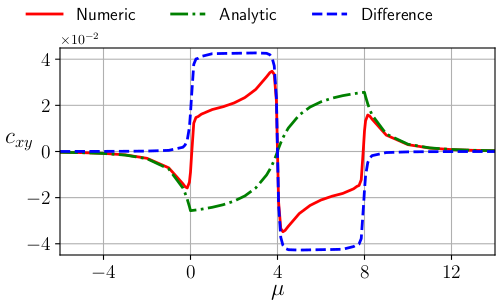#### Sidebar

News:
Follow me on twitter. (18/01/18)

Observing quantum physics with BigRed3
Homepage was renovated. (12/11/17)

Click here for more news…

Useful links:

en:projects

### Recent Research

How vortex bound states affect the Hall conductivity of a chiral px ± ipy superconductorThe tight-binding result of cxy is compared with the prediction of the effective low-energy theory of the $p$-wave superconductor.

This work extend our understanding of the anomalous charge response, cxy of chiral superconductors. It is established that in order to correctly apply the Streda formula for calculating cxy it is necessary to employ compact geometries that avoid edge effects. This, in turn, requires a careful analysis of the effect of finite-radius vortex nucleation that leads to an adjustment of the Streda formula. The modified Streda formula is then applied to calculate cxy for a px ± ipy superconductor placed on a square lattice at zero magnetic field and zero vorticity. We show that $c_{xy}$ is a sum of two contributions, one which is non-universal and the other equals $\kappa/8\pi$, where $\kappa$ is the Chern number of the superconductor. Moreover, we note that cxy is proportional to the anomalous Hall conductivity, which in turn is proportional to the polar Kerr angle. Thus, these results should affect the calculation of the polar Kerr effect, hence they are significant for the determination of the order parameter of superconductors.

Signatures of the topological spin of Josephson vortices in topological superconductors

Realization of non-abelian quasi-particles known as Majorana fermions is an ongoing challenge for physicists exploring topological states of matter. Towards achieving this goal, we recently suggested that Josephson vortices in topological Josephson junctions (TJJ) would constitute such Majorana fermions and retain the exchange statistics of bulk vortices. We corroborated this hypothesis by finding the universal exchange phase of Josephson vortices. In order to do so, we derived the Hamiltonian governing the dynamics of a soliton in an annular Josephson junction. Our next step was to develop a procedure to calculate the Berry connection of systems that posses particle-hole symmetry. The procedure was applied to confirm that the Abelian phase due to the an exchange between a vortex in the bulk of a p-wave superconductor and a Josephson vortex is π/8. In addition, we suggested an experiment to measure it by.

On the effective theory of vortices in two-dimensional spinless chiral p-wave superfluids

As the search for quantum computers evolves, new methods to realize an universal topological quantum computation are a explored. Vortex defects in a 2D spinless chiral p-wave superfluid bind Majorana zero modes that endow them with non-Abelian exchange statistics. Motivated by its potential for topological quantum information processing, we developed a ${\mathbb{U}(1) \times \mathbb{Z}_2}$ effective gauge theory for vortices in a ${p_x+ip_y}$ superfluid in two dimensions. The combined gauge transformation binds ${\mathbb{U}(1)}$ and ${\mathbb{Z}_2}$ defects so that the total transformation remains single-valued and manifestly preserves the particle-hole symmetry of the action. The ${\mathbb{Z}_2}$ gauge field introduces a complete Chern-Simons term in addition to a partial one associated with the ${\mathbb{U}(1)}$ gauge field. The theory reproduces the known physics of vortex dynamics such as a Magnus force proportional to the superfluid density. It also predicts a universal Abelian phase, ${\exp(i\pi/8)}$, upon the exchange of two vortices, modified by non-universal corrections due to the partial Chern-Simon term that are screened in a charged superfluid.

The role pickup ions play in the termination shock

An energy conservation paradox emerged from the data collected by Voyger 2 (V2) while crossing the Termination Shock in September 2013. The solar wind (SW) blows outward from the Sun and forms a bubble of solar material in the interstellar medium (ISM). The termination shock occurs where the SW changes from being supersonic to being subsonic. It was found that the sum of the flow energy and the thermal energy is not conserved. We showed that the pickup ions (PUI), a group of atoms from the ISM that are Ionized by the Sun's radiation and then swept along with the SW, which have an energy spectrum that lies outside the V2's equipment range of the measurement gain the missing energy. It was done with the use of the Liuoville theorem to map the PUI momentum distribution between the two sides of the shock. We found that: (a) the PUI can gain the missing energy and momentum along the shock with a Shock Drift Mechanism (SDM) (b) The SDM must be preliminary to the Shock.

en/projects.txt · Last modified: 2019/02/18 15:15 by ariad

### Page Tools